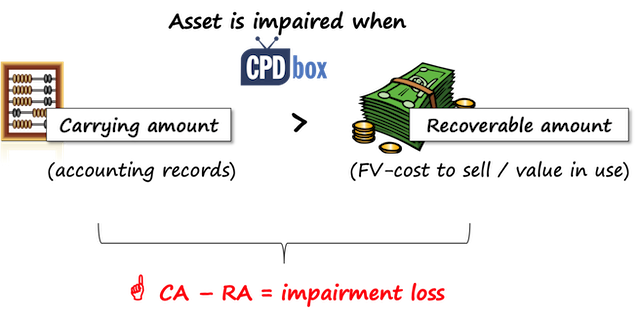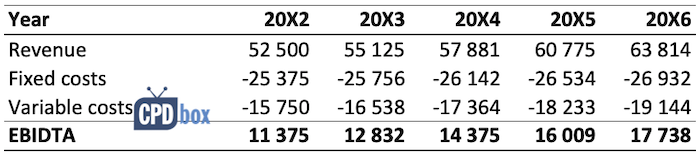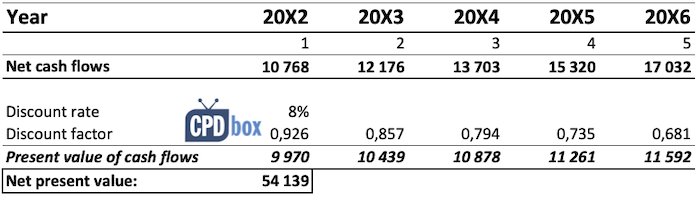In my last article, I tried to outline the main things to consider and to avoid when preparing the cash flow projections for the impairment tests under IAS 36.

Here let’s illustrate the theory and show the numerical example of making cash flow projections for impairment tests.

Please be warned that there are many variations of the cash flow projections and value in use calculations, and you should always apply your judgment (while following the rules).

And now the small recap:

The impairment testing aims at proving that the carrying amount of an asset or cash-generating unit is LOWER than its recoverable amount.Recoverable amount is the higher of fair value less costs of disposal and value in use.

We always know the carrying amount – please look to your financial statements or accounting records.

However, in order to determine the recoverable amount, we need to find out either fair value less costs of disposal or value in use.

## Single asset or cash generating unit?

The first decision you should make in your impairment test is:

Should you test a single asset or a cash-generating unit?

It depends.

You should test a single asset for impairment when:

• It is possible to determine the asset’s fair value less cost of disposal AND it is greater than the asset’s carrying amount. In this case, you don’t have to calculate value in use, because the asset is not impaired; or
• The asset generates individually identifiable and independent cash inflows.

Based my own experience, vast majority of companies perform impairment tests on cash-generating units, because it is hard to determine fair value of used assets and the assets generate independent cash inflows rarely on its own.

Special For You! Have you already checked out the  IFRS Kit ? It’s a full IFRS learning package with more than 40 hours of private video tutorials, more than 140 IFRS case studies solved in Excel, more than 180 pages of handouts and many bonuses included. If you take action today and subscribe to the IFRS Kit, you’ll get it at discount! Click here to check it out!

For this reason, this example will show you how to draft the cash flow projections for value in use calculation on cash-generating unit (CGU), not on an individual asset.

## Example: Cash flow projections for cash-generating unit

A parent performs an impairment test of its cash-generating unit, which is a whole subsidiary.

The carrying amount of a subsidiary, including allocated goodwill and working capital (current assets and current liabilities), is CU 150 000.

It is not possible to determine its fair value less costs of disposal and therefore the parent determines the subsidiary’s recoverable amount based on its value in use.

At the end of 20X1, the subsidiary reported the following results:

• Revenue from principal activities: CU 50 000
• Fixed costs: CU 25 000
• Variable costs: CU 15 000
• Net profit for the year before taxation: CU 10 000
• Closing working capital (net current asset): CU 1 000

For the purpose of value in use calculation, the parent made the following assumptions:

• Based on information about the subsidiary’s industry, the market expects the growth of 5% per year in average for the next 5 years, and then 2% in the long term. These rates are nominal and include the effect of inflation.
• Pre-tax discount rate determined based on company’s cost of capital is 8% p.a.
• Based on past experience, the company assumes the annual replacement capital expenditure of CU 500 each year net of the inflation effect.
• Fixed costs are the same in real terms (hint: careful about the inflation!). They exclude depreciation and amortization.
• Variable costs are about 30% of the revenue.
• The assumed inflation rate is 1.5% p.a. for the next 10 years.

### Step 1 – Starting point: 5-year forecast

When you are testing a whole company or even its part as CGU, it is necessary to draft net pre-tax cash flows generated by that CGU for a maximum of 5 years.

Our starting point is to prepare the forecast of CGU’s profits – not yet cash flows.

Based on the numbers and assumptions above, we can calculate the net profits as follows:Notes:

• Revenue in the year 20X2 was calculated as revenue in 20X1 of CU 50 000 increased by the growth rate of 5%: 50 000*(1+5%) = 52 500. Revenues in the next years were calculated accordingly.
• Fixed costs were increased by the inflation rate of 1.5% each year. For the year 20X2: CU 25 000*(1+1.5%) = 25 375
• Variable costs are simply 30% of revenue
• EBIDTA = earnings before interest, depreciation, tax and amortization

Now, you might ask: Why did we stop at profit before tax, depreciation, interest and amortization?

The reasons are as follows:

• Depreciation and amortization are not included, because they are NOT the cash items. Our aim is to arrive at pre-tax cash flows.
• Interest is a financing item which is excluded as required by IAS 36;
• Value in use is calculated on a pre-tax basis to avoid complications related to tax losses carried forward, deferred taxation, etc.

### Step 2: Adjust EBIDTA to arrive at cash flows

Once you have your EBIDTA forecasts, you need to realize that they do not represent the cash flow projections for value in use calculation.

Not yet.

We have to take a few more items into account:

• Changes in working capital: payments of liabilities and collections of receivables generally do not enter into EBIDTA, but they affect the net cash flows.
Therefore, don’t forget to adjust EBIDTA by the net change in working capital.
• There might be some expenditures for replacement of non-current assets in order to maintain the production capacity of CGU. Neither those are included in EBIDTA, because they enter in profit or loss as depreciation or amortization.

With regard to changes in working capital, you need to forecast the net balance of your working capital at the end of each period and then adjust EBIDTA.

For example, if opening net balance of your working capital in 20X2 is CU 1 000 (net asset) and closing balance of your working capital in 20X2 is CU 1 100, then the cash flow change in net working capital is CU -100.

The logic is simple: you have more money tied in a working capital at the end of 20X2 than in the beginning of 20X2, thus the net cash flow change is negative as you have less cash available (CU 1 000-CU 1 100).

Our adjusted cash-flow table is as follows:Notes:

• Opening and closing net working capital numbers are forecasted, in other words – I made them up in this example.
• Capex is assumed annual 500 increased by the inflation rate.

### Step 3: Discount net cash flows with pre-tax discount rate

Now it’s time to select the most appropriate discount rate.

In many cases, companies use their own cost of capital derived by WACC model, which is often OK for auditors, too.

However, be careful, because WACC will give you post-tax rate and you are required to use pre-tax rate here.

You can make an attempt to calculate value in use based on post-tax rate, but in such a case your cash flows need to incorporate tax effects – and that is not a very nice, neat and reliable exercise.

I strongly recommend calculating pre-tax rate from your post-tax rate (e.g. WACC)here’s an article that can help.

However, let me add here, that this formula not always works well. In other words, pre-tax rate is not always the post-tax rate grossed up by the appropriate tax rate.

But let’s not get into many details here, because that’s the topic for another article.

One more consideration when discounting:

Special For You! Have you already checked out the  IFRS Kit ? It’s a full IFRS learning package with more than 40 hours of private video tutorials, more than 140 IFRS case studies solved in Excel, more than 180 pages of handouts and many bonuses included. If you take action today and subscribe to the IFRS Kit, you’ll get it at discount! Click here to check it out!

You need to make a good assumption when most of your cash flows happen.

In the beginning of the year? At the year-end? Or are they spread evenly throughout the year?

If they are spread evenly, then you should apply so-called “mid-year convention” in calculating your discount factors.

For example, let’s say that you want to discount cash flows in the year 2 and you assume they are spread evenly throughout the year 2.

Thus you will use the number 1.5 as number of years in the discount factor formula. It indicates, that the cash flows happen in the middle of the year 2.

In the following table, I used the year-end convention. For example, in the year 2, I used number 2 as number of years in the discount factor formula:Notes:

• Discount factor for the year 20X3 (year 2) = 1/((1+8%) to the power of 2)
• Present value of cash flows in the year 1 = net cash flows in the year 1 of 10 768 multiplied with the discount factor for the year 1
• Net present value = sum of all present values of cash flows in individual years

### Step 4: Calculate terminal value

As I explained in the previous article, terminal value is a very important number because it estimates the net cash flows beyond forecasted period of maximum 5 years.

In some cases the terminal value represents the net cash flows to receive from the sale of an asset at the end of its useful life.

However, in this particular case we can reasonably assume that a subsidiary – our CGU in testing – will operate its business for indefinite number of years.

Therefore the terminal value estimates the net cash flows beyond forecasted period.

Two most common methods to calculate it are:

1. Exit multiple – this is the multiple of shareholders’ cash flows in the last year of projections.
In our example, we assume to make net cash inflow of 17 032 CU at the end of year 5 in your projections.
Market data say that similar companies sell for multiple of 10 meaning that to sell your company you can ask ten times your net shareholders’ cash flow.

In this case, you can estimate your terminal value to be 10 x 17 032 = 170 320.

Then you need to discount it with the pre-tax discount rate: 170 320 * 0,681 = 115 988 and include in the cash flow projections.

2. Perpetuity – you would take the last year’s projection and apply perpetuity formula to it.
In this example, we projected the net cash inflow of 17 032 CU at the end of year 5.
We assume the long-term growth rate to be 2% and your pre-tax discount rate is 8%.
Then we can apply the growing perpetuity formula which is the cash flow after the first period divided by the difference between the discount rate and the growth rate.
In fact you are calculating growing perpetuity as a series of periodic payments that grow at a proportionate rate for an infinite amount of time.
So, your terminal value would be:

• CU 17 032, being the net cash flow in the last year (year 5) – just note that here it is unadjusted, but you should adjust it for terminal value calculation if needed;
• Increased by the annual rate of 2%: CU 17 032*(1+2%)= 17 373;
• Divided by the difference between the discount rate of 8% and growth rate of 2% = 17 373/(8%-2%) = 289 550;
• Discounted to present value, because you calculate this at the end of year 5 = 289 550 * 0,681= 197 184

As you can see, the two amounts are different when we used different methods:

• Exit multiple method: CU 115 988; and
• Perpetuity method: CU 197 184.

This is quite normal, because perpetuity method assumes to carry on with business and accept business risks beyond 5 years, and exit multiple method assumes selling the business and getting rid of all associated business risks.

Which method to apply?

When the plan is to sell a subsidiary after 5 years, then exit multiple is probably more suitable method.

However, when the plan is to continue the business indefinitely, then perpetuity method is more acceptable.

### Step 5: Sum up and calculate value in use

To arrive at value in use, we must sum up the net present value of cash flows in the next 5 years and the present value of terminal value:

• Net present value of cash flows: CU 54 139
• Present value of terminal value (using perpetuity method): CU 197 184
• Value in use: CU 251 323

You can now see why you should get the terminal value right: it represents 78% of value in use.

The carrying amount of CGU was CU 150 000. Value in use is CU 251 391, which is much greater than the carrying amount and thus there’s no impairment.

## Final word

Please – if you calculate your value in use very close to the carrying amount, then expect that your auditor will ask a lot of questions and examine your calculations thoroughly.

The reason is that in such a sensitive case, even a slight shift in your assumptions can bring you from “no impairment” to “impairment loss like a hell”.

Did you like this article or do you have any question? Please leave me a comment below. Thanks!